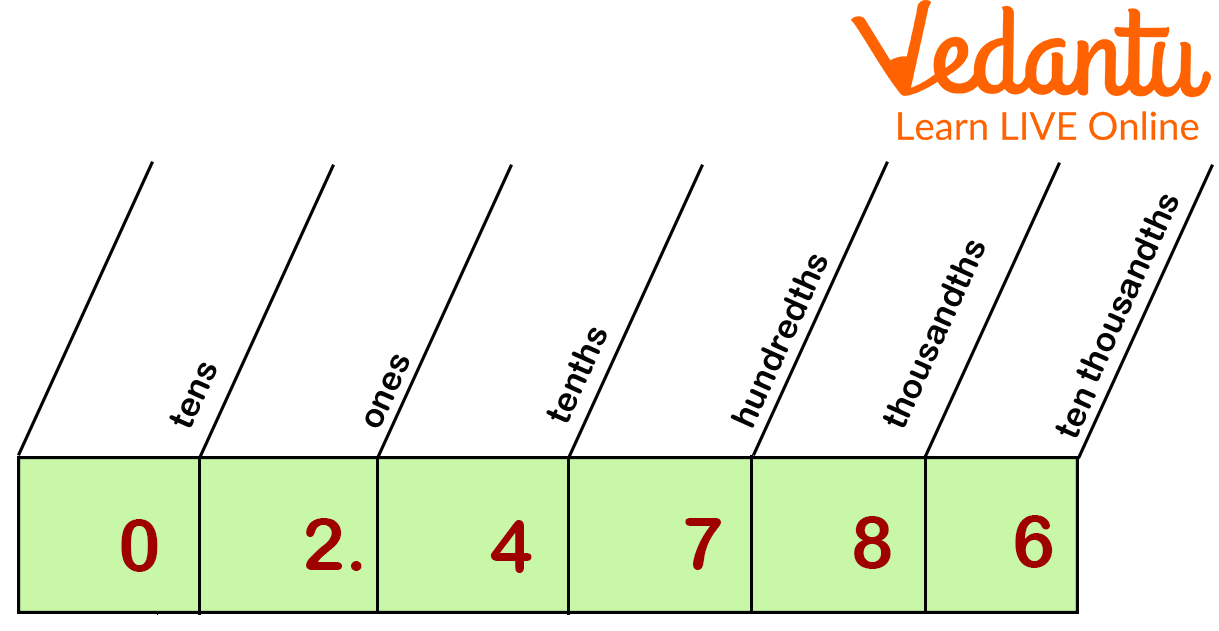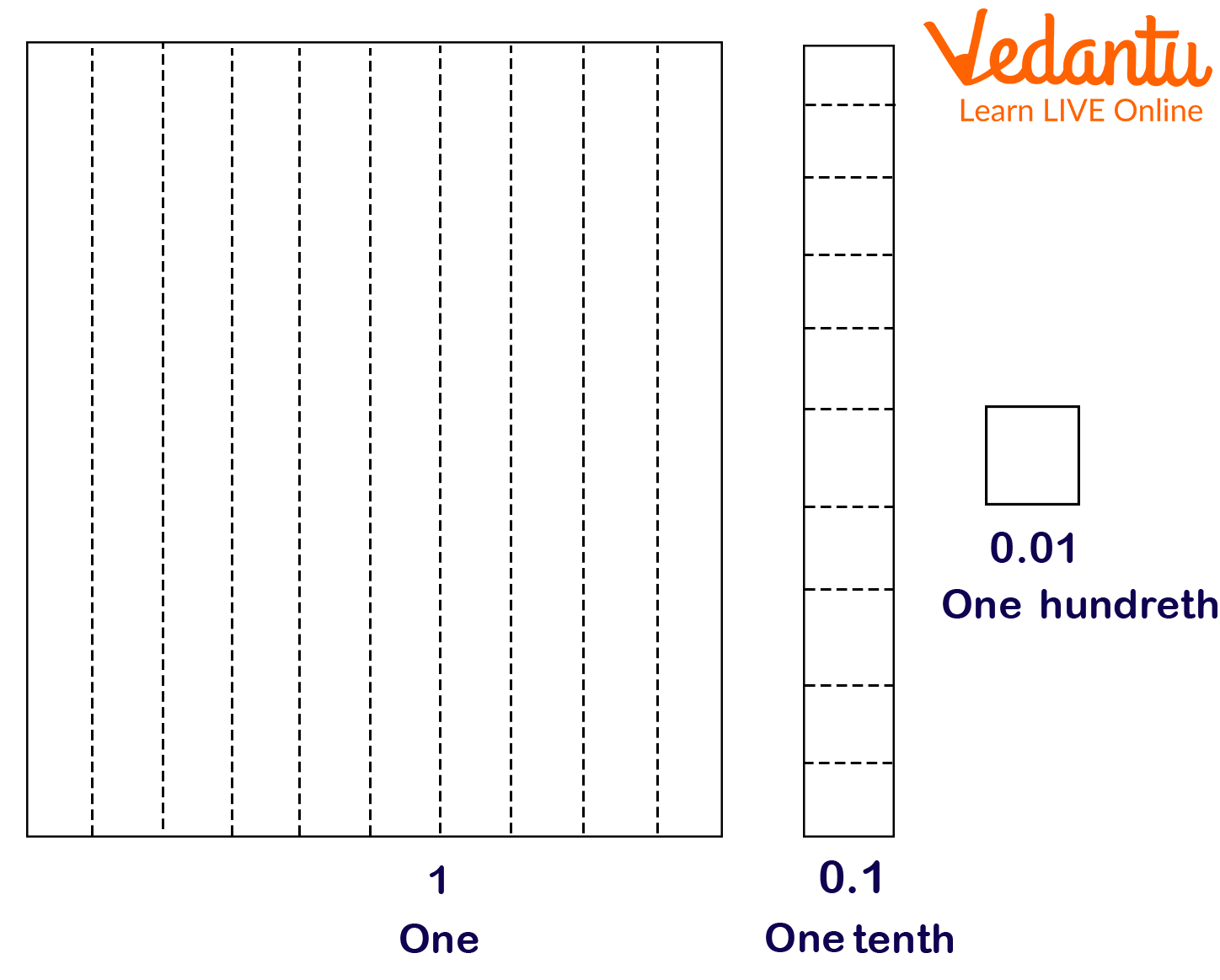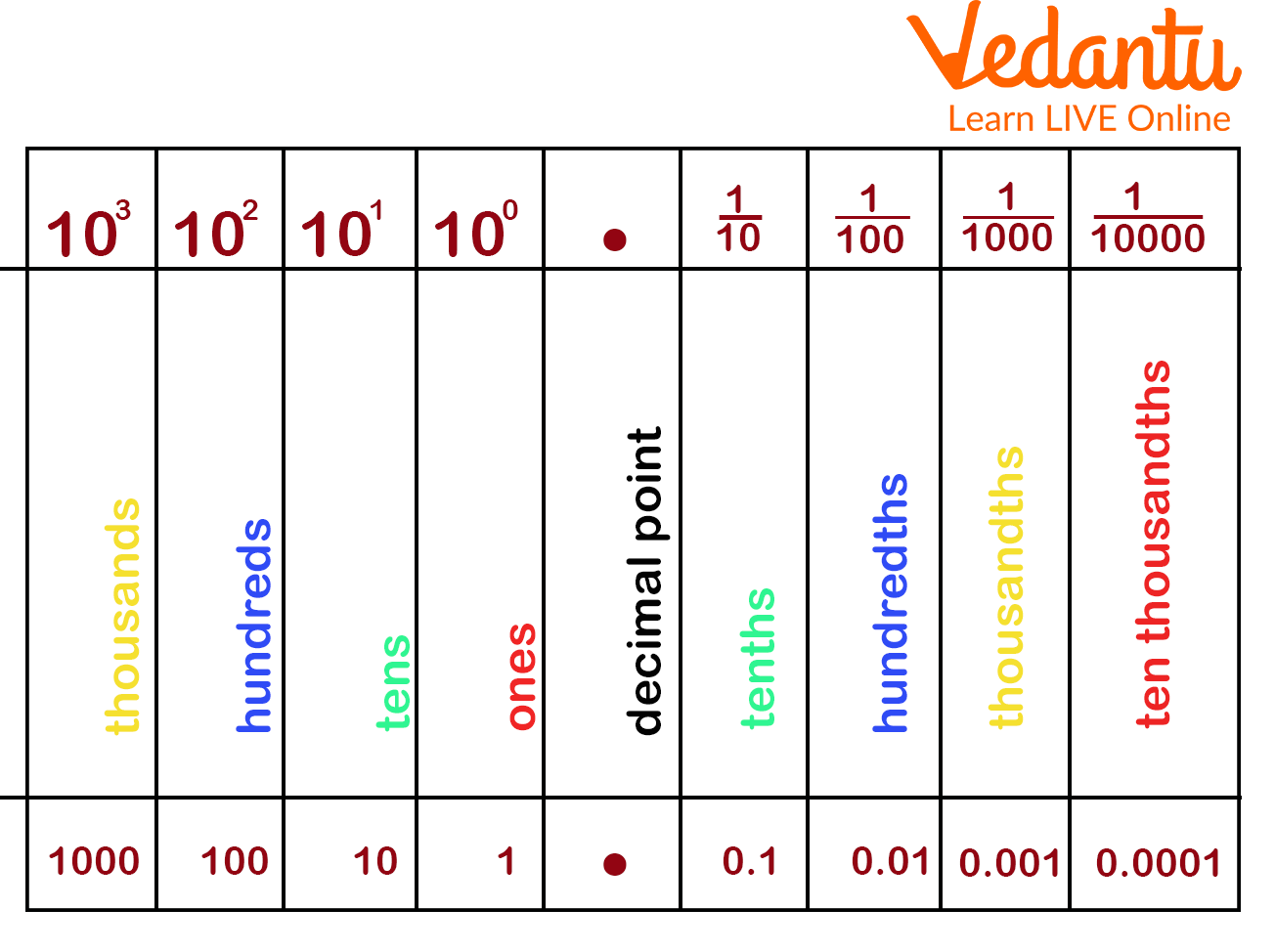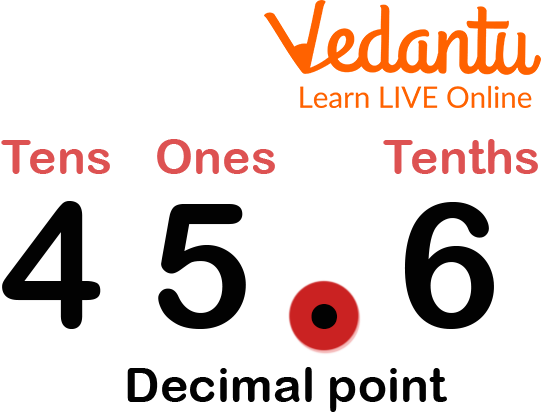Courses
Courses for Kids
Free study material
Free LIVE classes
More

# Tenth Decimal PlaceLIVE
Join Vedantu’s FREE Mastercalss

## Introduction to Tenth Decimal Place

Decimals have always been a difficult topic for students. A decimal number comprises a whole number, and a fractional part that is separated by a dot called the decimal point. For example, 5.37 is a decimal number 5 is the whole number part, and .37 is the fractional part. The tenth decimal place, or decimal point, is the digit immediately to the right of the decimal separator with a value of 0.1. For example, if you have a number with one decimal place - such as 2.1 - then the tenth decimal place would be 1. The remaining ones continue to fill in the place values until there are no digits.

## Tenth Decimal Place

The "deci-" prefix, as in "decimeter," denotes the tenth place. A decimeter is one-tenth of a meter. Now the question arises where is the tenth place in a decimal? According to the place value chart, the tenth place is situated between the decimal point and the hundredth place.Place Value

## Visualization of Tenth Decimal Place

Using base 10 blocks is useful for visualizing decimals. Consider a huge square representing one whole, for example. Each of the 10 equal-sized strips created from the square symbolizes one-tenth, or 0.1. To represent hundredths, each strip can be divided into 10 smaller squares.Visualisation of Tenth Decimal Place

## What is the Tenth Place in a Decimal?

In a decimal number, as we move to the left, taking decimal as a reference point, each position is 10 times bigger. If we move to the right from the decimal point, each position is 10 times smaller. For example In, the number 396.7

• The "6" is in the One's position, meaning 6 ones (which is 6).

• The "9" is in the Tens position meaning 9 tens which are ninety.

• And the "3" is in the Hundreds position, meaning 3 hundred.

This is when we move left from the decimal point; when we move right to the decimal point, each position is 10 times smaller, and tenths are $\dfrac{1}{10}$. For example, 396.7; the number will be read as three hundred ninety-six and seven-tenths, but we usually read it as three hundred ninety-six point seven.

Let’s explore where is Tenth Place in a decimal. The placement (or "place") of each digit in a number is crucial. The digit following the decimal point should be read with the word "tenths" in place of the decimal point. The alternative reading strategy is to just read the decimal point as a "point" before reading each individual digit as a word.Location of Tenth Place in Decimal

The tenth place, as well as the adjacent place values, are displayed in red. Any digit that is in the tenth place has a value of 0.1, which is the result of the digit and $\dfrac{1}{10}$. For instance, the digit in the tenth place in the number 5.62 is 6, and its value is $0.1 \times 6 = 0.6$, or $\dfrac{6}{10}$.

## Tenth Decimal Place Examples

let's have an example:

Here is the decimal representation of the number "forty-five and six-tenths":

Between Ones and Tenths, the decimal point is located.Tenth Decimal Place

4 Tens, 5 Ones, and 6 Tenths make up the number 45.6 as follows:

$45.6 = 40+5+\dfrac{6}{10}$

## Solved Examples

Q1. What is the sum of the digits at the tenth and hundredth places in the number 344.768?

Ans: Here, the digit at the tenth place = 7

The digit at the hundredths place = 6

Therefore, the Sum of digits= 7+6 = 13.

Q2. What number appears in the tenth place in 93.347?

Ans: The first digit following the decimal point is represented by the tenth place.

The second digit following the decimal point in 93.347 is 3.

Q3. What is 2.3?

Ans: The entire number portion, "2," is located on the left.

The number three is in the "tenths" position, which is "3 tenths," or $\dfrac{3}{10}$.

2.3 is, therefore, "2 and 3 tenths."

## Practice Problems

Q1. Find the tenth-place value in 8.364. (Ans: 3)

Q2. Find the tenth-place value in 1058.56. (Ans: 5)

## Summary

Decimals provide a convenient shorthand for fractions and mixed numbers with denominators that are powers of 10, such as 10,100,1000,10000, etc. The first digit represents the tenth place following the decimal. If a number has one, the first digit to the right of the decimal point represents the number of tenths. The second digit represents the number of hundredths to the right of the decimal point. For instance, the fractions $\dfrac{3}{10}$ and the decimal 0.3 are equivalents.

Last updated date: 17th Sep 2023
Total views: 93.6k
Views today: 2.93k

## FAQs on Tenth Decimal Place

1. How can a place value chart for decimals be helpful to students?

The basis for regrouping, multiple-digit multiplication, and other operations in the decimal system is established by place value, which also acts as a platform for comprehending other base systems. It serves as the basis for regrouping and enables us to compare numbers, arrange them vertically, and understand addition, subtraction, multiplication, and division with greater numbers. A decimal place value chart can be used to compare and organize decimals and determine the place values of decimal digits.

2. How are decimal places rounded to the tenth?

Look at the hundredth number to round the decimal number to the nearest tenth. If it's more than 5, add one to the tenth value. Remove all the numbers that are present after the tenth place and leave the tenth place value alone if it is less than 5. To round to the nearest tenth, for example –, look to the right of the tenth place digit, which is the hundredth place digit. You then get to add one to the tenth digit if the result is 5 or higher. The tenth digit stays the same if it is 4 or less.

3. Can any digit have the same place value and face value?

A number's digits have distinct place values and face values apart from the one’s place. For instance, in the number 23.15, the place value of 2 is 20, while its face value is 2. The place value and face value of any digit provided in the unit place will always be the same. Aside from that, the place value and face value of 0 will never change.

However, the face value and place value of 3, which is in the ones place, are the same: 3.